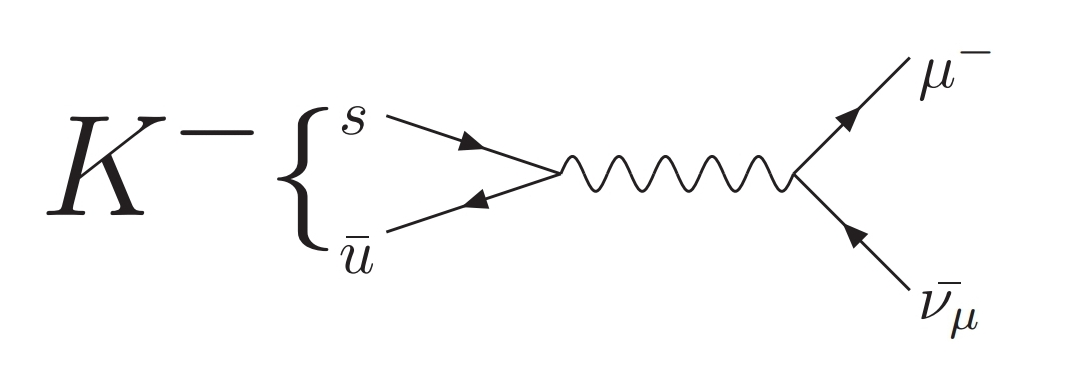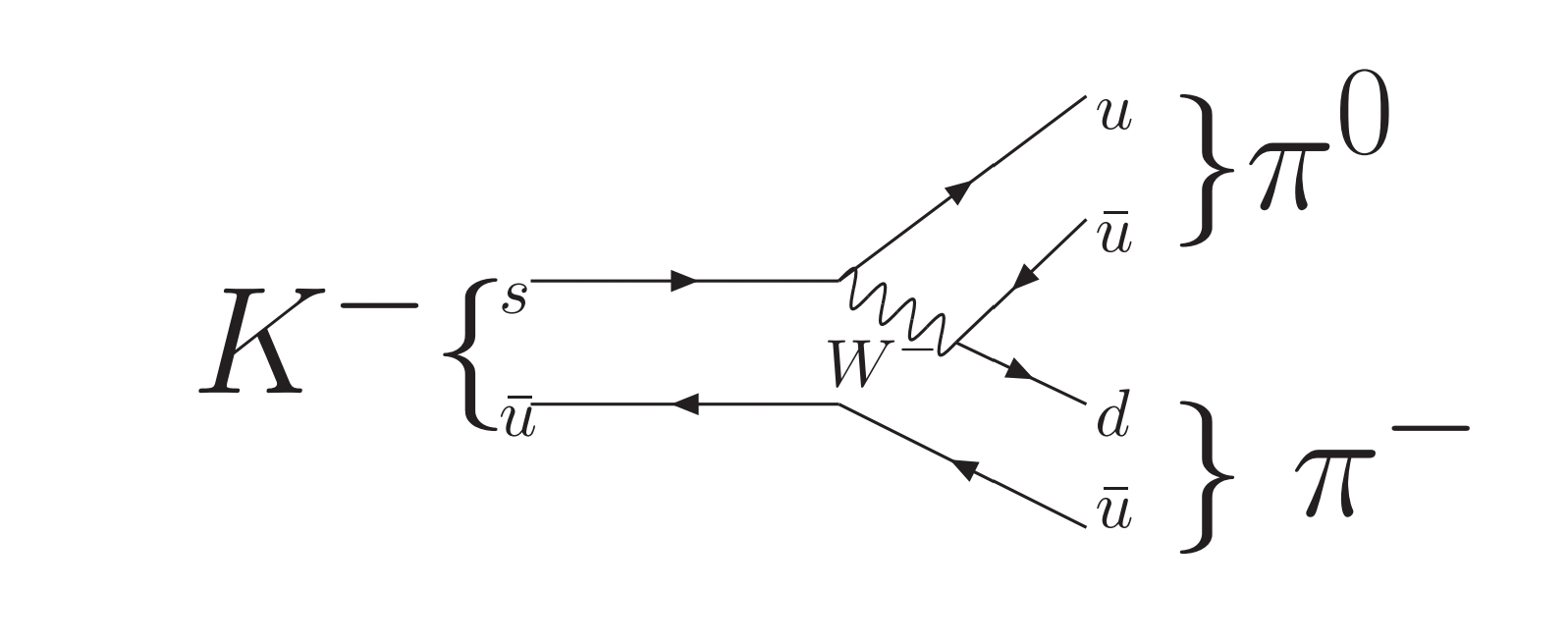# nLab leptonic decay

Contents

### Context

#### Fields and quanta

fields and particles in particle physics

and in the standard model of particle physics:

force field gauge bosons

scalar bosons

flavors of fundamental fermions in the
standard model of particle physics:
generation of fermions1st generation2nd generation3d generation
quarks ($q$)
up-typeup quark ($u$)charm quark ($c$)top quark ($t$)
down-typedown quark ($d$)strange quark ($s$)bottom quark ($b$)
leptons
chargedelectronmuontauon
neutralelectron neutrinomuon neutrinotau neutrino
bound states:
mesonslight mesons:
pion ($u d$)
ρ-meson ($u d$)
ω-meson ($u d$)
f1-meson
a1-meson
strange-mesons:
ϕ-meson ($s \bar s$),
kaon, K*-meson ($u s$, $d s$)
eta-meson ($u u + d d + s s$)

charmed heavy mesons:
D-meson ($u c$, $d c$, $s c$)
J/ψ-meson ($c \bar c$)
bottom heavy mesons:
B-meson ($q b$)
ϒ-meson ($b \bar b$)
baryonsnucleons:
proton $(u u d)$
neutron $(u d d)$

(also: antiparticles)

effective particles

hadrons (bound states of the above quarks)

solitons

in grand unified theory

minimally extended supersymmetric standard model

superpartners

bosinos:

dark matter candidates

Exotica

auxiliary fields

# Contents

## Idea

In the context of quantum hadrodynamics, the decay-mode of a meson via the weak interaction (i.e. by exchange of a W-boson or Z-boson) into a pair of leptons is called (shown are Feynman diagrams of example decays of kaons):All this is in contrast to

• non-leptonic decays where other mesons but no leptons are produced:### General

• L. G. Landsberg, Electromagnetic decays of light mesons, Physics Reports Volume 128, Issue 6, November 1985, Pages 301-376 (doi:10.1016/0370-1573(85)90129-2)

• R. Casalbuoni, A. Deandrea, N. Di Bartolomeo, F. Feruglio, R. Gatto, G. Nardulli, Phenomenology of Heavy Meson Chiral Lagrangians, Phys. Rept. 281:145-238, 1997 (arXiv:hep-ph/9605342)

#### Purely leptonic decays

• Tome Anticic, Purely leptonic decays of heavy flavor mesons 1997 (spire:454066)

### Leptonic decays of pions

Discussion of leptonic decays of pions:

#### General

• Dinko Pocanic, Emil Frlez, Andries van der Schaaf, Experimental study of rare charged pion decays, Journal of Physics G: Nuclear and Particle Physics, Volume 41, Number 11 (arXiv:1407.2865)

#### Purely leptonic decays

See at vector meson dominance.

• Rene Unterdorfer, Hannes Pichl, On the Radiative Pion Decay, Eur. Phys. J. C55:273-283, 2008 (arXiv:0801.2482)

• V. Bolotov, The radiative pion decays (pdf)

### Leptonic decays of $\omega$-mesons

Discussion of leptonic decays of omega-mesons:

• I. N. Mishustin, L. M. Satarov, H. Stoecker, W. Greiner, Dilepton production by bremsstrahlung of meson fields in nuclear collisions, Phys. Rev. C57:2552-2558, 1998 (arXiv:hep-ph/9709384)

• Anastasiya Kuzmenko et al. , The Study of the Conversion Decays of Omega Meson to $\pi^0$ Meson and $e^+ e^-$ Pair with the CMD-3 Detector, CERN Proceedings Archives Vol 1 (2017) (doi:10.23727/CERN-Proceedings-2017-001.91

### Leptonic decays of B-mesons

Discussion of leptonic decays of B-mesons:

#### Fully leptonic decays

• M. Lattery, Fully Leptonic Decays of B Mesons, 1996 (spire)

#### Semileptonic decays

• J. P. Lees et al. (BABAR Collaboration), Measurement of the $B^+ \to \omega \ell^+ \nu$ branching fraction with semileptonically tagged B mesons, Phys. Rev. D 88, 072006 (2013) (doi:10.1103/PhysRevD.88.072006)

• Xian-Wei Kang, Tao Luo, Yi Zhang, Ling-Yun Dai, Chao Wang, Semileptonic $B$ and $B_s$ decays involving scalar and axial-vector mesons, Eur. Phys. J. C 78, 909 (2018) (doi:10.1140/epjc/s10052-018-6385-9)

### In relation to flavor anomalies

Discussion of leptonic decays in view of observed flavour anomalies:

• N. Penalva, E. Hernández, J. Nieves, Hadron and lepton tensors in semileptonic decays including new physics (arXiv:2004.08253)

• N. Penalva, E. Hernández, J. Nieves, $\bar B_c \to \eta_c$, $\bar B_c \to J/\psi$ and $\bar B \to D^{(\ast)}$ semileptonic decays including new physics (arXiv:2007.12590)